# How to drastically reduce the cost on Google BigQuery by carefully choosing your data types

Google sales his Big Query based on a Pay by Use business model.

I assume that you will use big Query for what it is designed for, I mean a lot of data (billions of lines) reads several times a day during batches, calculation, aggregation or reports reads

As you pay by use, you need to pay attention to the types you are going to use.

For example, to store numbers Google will invite you to use NUMERIC types.

Let’s dive in the Google documentation: you have 3 types of numerics

• NUMERIC: 16 bytes
• FLOAT64: 8 bytes
• INT64: 8 bytes

I made a POC about two different types in order to check about elapsed time, IOs and CPU consumption

• I created 2 tables with a payment amount column (PAYMENT_AMT)
• In F_SALES_TRX_LINE_PAYMENT_LOAD_ETL_TYPES table I defined it as a FLOAT64
• In F_SALES_TRX_LINE_PAYMENT_LOAD_ETL table I defined it as a NUMERIC
• I loaded the table with the exact same data and the same number of records

Here is the result!

# Comparing the duration

## FLOAT64 Type

```select   sum(PAYMENT_AMT)
-- 1.4466987007999676E8
```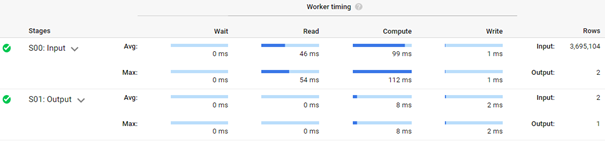## NUMERIC Type

```select   sum(PAYMENT_AMT)
--144669870.08
```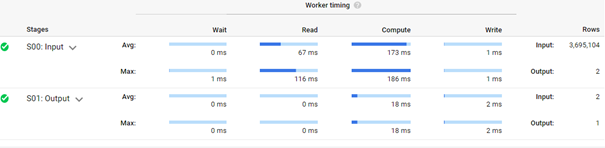## Duration analysis

Duration is almost the double for Numeric (258 – 153)

# Money costs

## FLOAT Type costs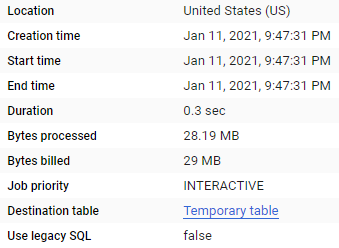## NUMERIC Type costs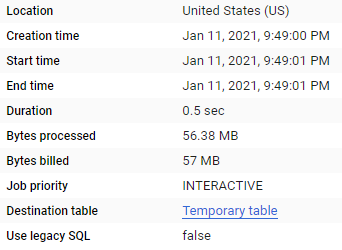Google will charge the double amount to perform the same calculation and obtain the exact same result.

57Mb will be charged for NUMERIC, vs 29Mb for FLOAT64

## Naturally losing precision on operations

Float implies some differences according to  IEEE.
If you execute

```SELECT
4.35 * 100 a_float
, CAST(4.35 AS NUMERIC) * 100 a_numeric
```

You will obtain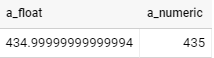## Workaround

A good workaround that will not imply any cost if to ROUND the float

Just put the right precision within the ROUND function

```SELECT
4.35 * 100 Regular_Float
, ROUND(4.35 * 100) Rounded_float
, CAST(4.35 AS NUMERIC) * 100 Regular_numeric
```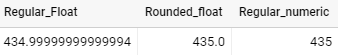```SELECT
(4.35 * 9.3  )* 100 Regular_Float
, ROUND((4.35 *9.3  ) * 100) Rounded_float
, ROUND( ((4.35 *9.3  ) * 100) ,2 ) Rounded_float_2
, CAST((4.35 *9.3 ) AS NUMERIC) * 100 Regular_numeric
```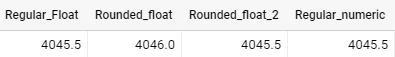# Conclusion

By choosing the right data types, you will